# 中国式一页纸矩阵表报告制作解析

## 什么样的叫中国式报表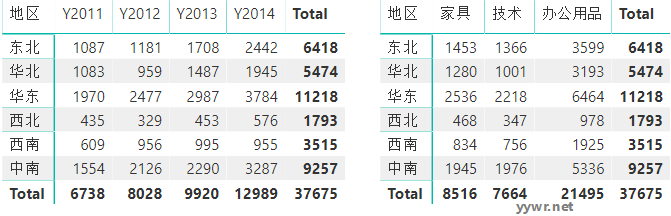## 如何在Power BI中实现中国式报表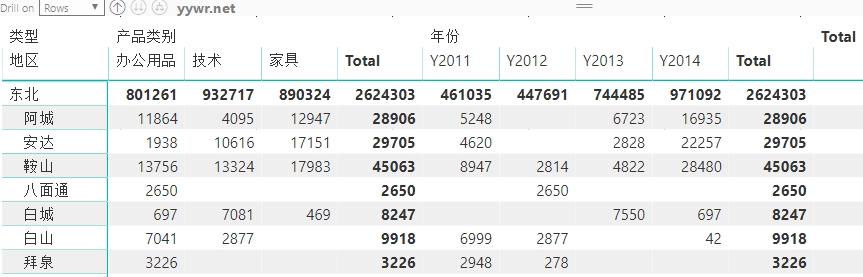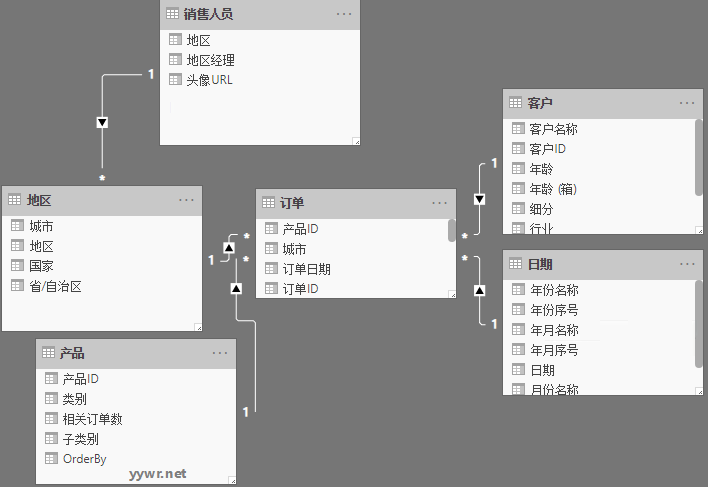## Power BI中国式报表简单版实现步骤

### 第一步，建立混合表头（中国式报表表头）

``````NewTable =
Return UNION(t_Year,t_Catalog)
``````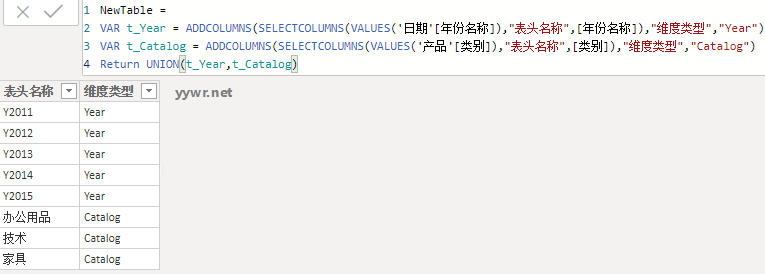### 第二步，建立计算度量值

``````SaleMeasure =
SWITCH(
TRUE(),
SELECTEDVALUE(NewTable[维度类型])="Year",
CALCULATE(SUM('订单'[销售额]),TREATAS(VALUES(NewTable[表头名称]),'日期'[年份名称]) ),
SELECTEDVALUE(NewTable[维度类型])="Catalog",
CALCULATE(SUM('订单'[销售额]),TREATAS(VALUES(NewTable[表头名称]),'产品'[类别]) )
)
``````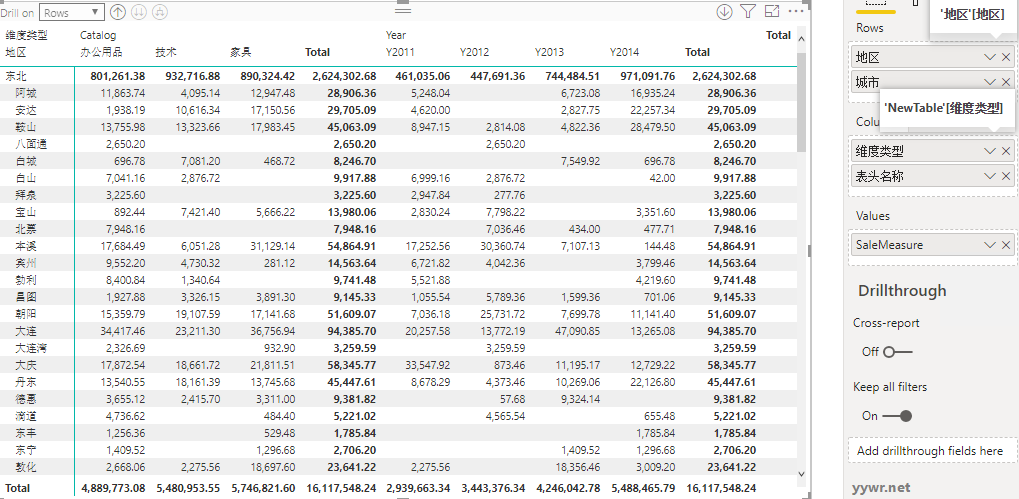## 总结

• https://www.jianshu.com/p/c8770edeba77
• https://blog.csdn.net/qq_44794714/article/details/88833667